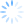# 慢羊羊的空间``````// 程序名称：圆弧组成的图案
// 编译环境：Visual C++ 6.0，EasyX 2011惊蛰版
// 最后修改：2011-3-7
//
#include <graphics.h>
#include <conio.h>
#include <math.h>

#define PI	3.1415926536
#define R	200

// 四舍五入
int Round(double x)
{
return (int)(x < 0 ? x - 0.5 : x + 0.5);
}

// 主函数
void main()
{
// 变量定义
int x, y;	// 坐标
double a;	// 弧度

// 绘图环境初始化
initgraph(640, 480);	// 初始化图形窗口
setorigin(320, 240);	// 设置坐标原点
setcolor(GREEN);		// 设置绘图颜色

// 绘图
circle(0, 0, R + 1);
for (int i=0; i<126; i++)
{
if ((i % 21) < 7)
{
a = PI * 2 * i / 126;
x = Round(cos(a) * R);
y = Round(sin(a) * R);
arc(x, y, PI * 2 / 3 - a, PI * 4 / 3 - a, R, R);
}
}

// 按任意键退出
getch();
closegraph();
}

``````